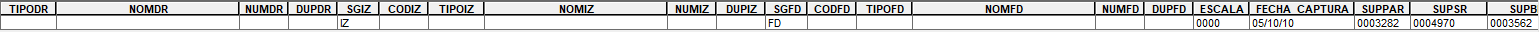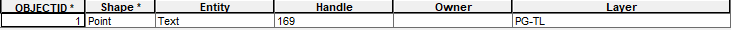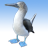# CalculateField_management ERROR, I can't understand why

313
1
10-02-2017 04:03 AMNew Contributor

Hello friends,

I'm having troubles with CalculateField_management in a python script (arcpy). I'm getting this error:

arcgisscripting.ExecuteError: ERROR 000539: Invalid field ASCFxCC.CODVIA

This is my code:

```GDBEntrada = "C:\data\mygdb"
expresion = 'funcion( !ASCFxCC.CODVIA!, !ASCFxCC.NUMPOL!, !ASCFxCC.DUPLICADO!)'
arcpy.CalculateField_management(AnnoCalle_Layer__2_, "Handle", expresion, "PYTHON_9.3", "def funcion (a,b,c):\\n  x='0'\\n  v='00000'\\n  n='0000'\\n  d=0\\n  if a != \"     \":\\n   v=a.lstrip('0')\\n   if b != '    ' :\\n     n=b.rstrip(' ')\\n     n=n.lstrip('0')\\n     if c != ' ':\\n       d= ord(c)\\n   x= v + n + str(d)\\n   return int (x)")```

This is table ASFxCC in mygdb :And this is the AnnoCalle_Layer__2_Why I'm getting this ERROR ?

Best,

Alberto.by
Occasional Contributor III

If you manually calculate the field inside arcmap, you can then copy as a python snippet the required code using the ArcGIS Results window.

Geoprocessing --> results

I guess you may need code like unless your data is joined:

```GDBEntrada = "C:\data\mygdb"